Times Tables - Definition with Examples

The Complete K-5 Math Learning Program Built for Your Child

• 30 Million Kids

Loved by kids and parent worldwide

• 50,000 Schools

Trusted by teachers across schools

• Comprehensive Curriculum

Aligned to Common Core

Times Tables

A times table is a list of multiples of a number. For instance, if we want to work out the times table for 2, we start with 2 and then add 2 in each step. The answer obtained in every step is a multiple of 2 and is known as multiplication fact.

Some of the times tables are illustrated below:

Times Table of 2:

 Times Table (2) Meaning Illustration 2×1=2 1 lot of 2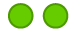2×2=4 2 lots of 2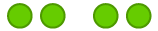2×3=6 3 lots of 2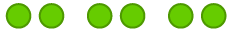2×4=8 4 lots of 2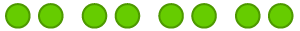We can find out the times table of other numbers also.

Example 1: Find out the times table of 5.

To work out times table of 5, we add  5 each time.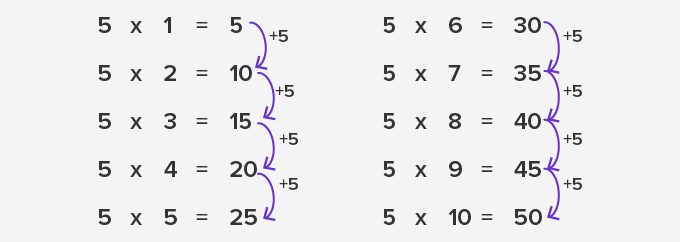Example 2: Find out what can we write in place of question mark?

7×3=?

7 times 3 mean 3 lots of 7 or 7 lots of 3.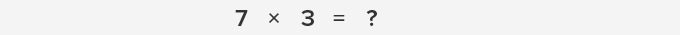Or we can work out in another way,Example 3: Find out the missing number.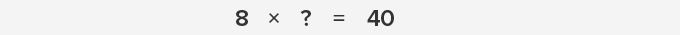It means some lots of 8 give us 40. So we can use division fact here.Hence our answer is 5.

 Fun Fact- In the times table of 9, each number makes a sum of 9.

Won Numerous Awards & Honors